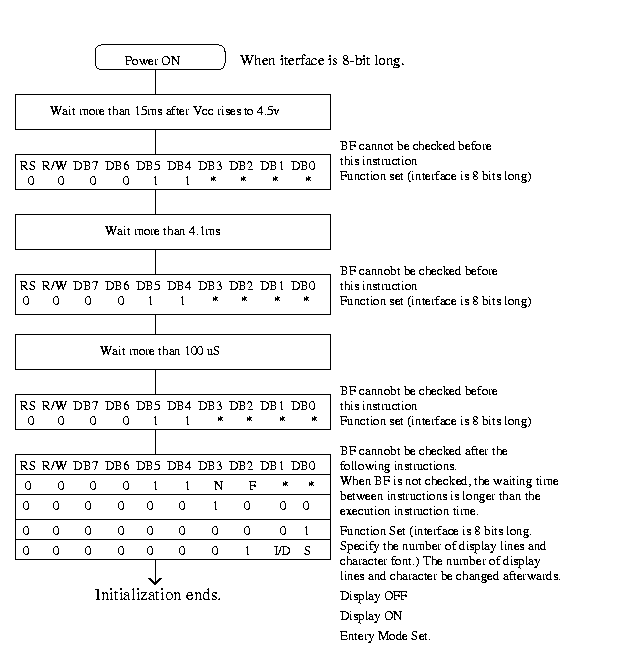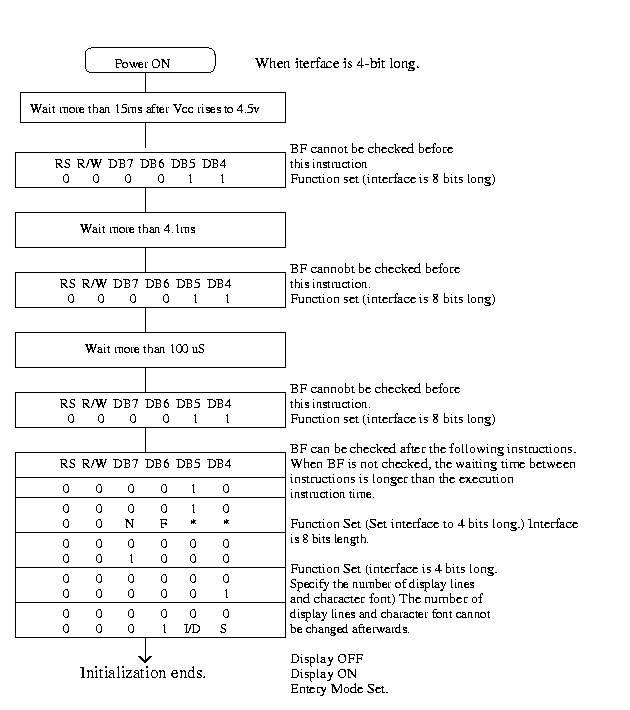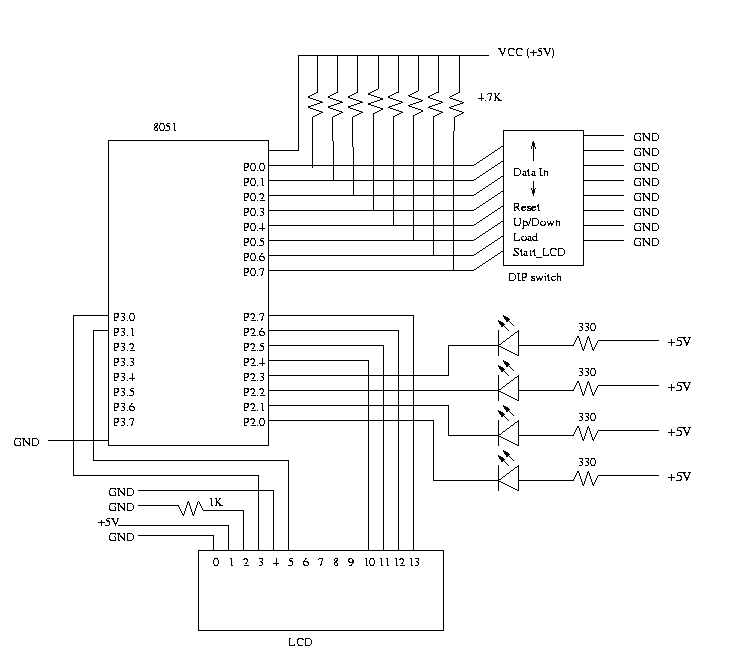Interfacing: Converting 8-bit LCD communication to 4-bit

Introduction

One of the challenges an embedded systems designer must face is the limited number of output pins on a microprocessor, in the case of the 8051 we are limited to 32. If we have a large number of peripherals connected to the 8051 we might not have the luxury of using 8 pins to interface to the LCD. The Optrex LCD has two modes, an 8-bit interface and a 4-bit interface. In this lab we will implement the 4-bit LCD interface.

Before an LCD can be used it must be initialized. Both the 8-bit and 4-bit initialization sequence is listed below. The following figures provide the contents of the Optrex databook for the LCD. You can also print these out here     8-bit interface     4-bit interface.Code Description Executed Time(max.)
 I/D=1: Increment DL=0:4-bit I/D=0: Decrement N=1:2 Rows S=1: With display shift N=0: 1 Row S/C=1: Display Shift F=1:5x10 dots S/C=0: Cursor movement F=0: 5x7 dots R/L=1: Shift to the right BF=1 : Internal operation is being performed R/L=0: Shift to the left BF=0: Instruction acceptable DL=1: 8-bit
DDRAM: Display Data RAM
CGRAM: Character Generator RAM
AC: Address Counter, used for both DDRAM and CGRAM
*: Invalid
fcp or fosc=250kHz
However, when frequency changes, execution time also changes

Ex
If fcp or fosc is 250kHz, 37µS x (270/250)=40µS

Assignment

1. Convert the io.c and io.h files to reflect an 4-bit encoding.
2. We are assuming that the remaining 4 pins are connected to peripherals so these pins should not change when we initialize and send data to the LCD.

Appartus Required

1. 8051
2. LCD
3. 4.7 K resistor (8)
4. 1 K resistor
5. 330 Ohm resistors (4)
6. LED (4)

SchematicProcedure

1. Wire up the circuit as shown in the schematic.
2. Download all the original 8-bit interface files. Some modification have been made to keep it consistent with the initialization sequence. Do not use code from previous labs, it may not match.
3. Copy your main.c file from Lab 1. No changes to this code should be required.
4. Modify the io.h and io.c files to convert the 8-bit interface code to the 4-bit interface.
5. Modify your main() code such that before it perform any LCD operations, it executes the following command:

`        P2 = 0;`
The four LED's connected to the lower 4 bits of P2 should always remain on. You must ensure that the lower 4 bits of P2 do not change, as these pins may be necessary to control other aspects of an embedded system. Thus, if your modifications are correct, the LCD should function properly and the LED's should not blink.
6. Follow the instructions given in Lab 1 to map your network drive, edit, compile and run your program using PDS51.
7. In your lab report, be sure to explain in detail the difference between the 8-bit and 4-bit LCD protocol.